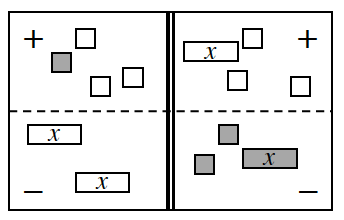### Home > CC3 > Chapter 4 > Lesson 4.1.1 > Problem4-9

4-9.

Write the equation represented by the diagram at right.

1. Simplify as much as possible and then solve for $x$.

Combine like terms

Move the variable to one side of the equation.

$1+(−3)+2x=(−x)+(−3)+(−2)+(−x)$

$−2+2x=−2x+(−5)$

$−2+2x=−2x+(−5)$
$+2\ \ \ \ \ \ \ \ \ \ \ \ \ \ \ \ \ \ \ \ \ \ \ +2$

$2x=−2x+(−3)$

$2x=−2x+(−3)$
$+2\ \ \ \ \ \ \ \ \ \ \ \ \ \ \ \ \ +2$

$4x=−3$

$x=−0.75$

Insert the number you got for $x$ into your original equation to make sure it is correct.